# 23.1.12. Vector analysis¶

## 23.1.12.1. Basic statistics for fields¶

Generates basic statistics for a field of the attribute table of a vector layer.

Numeric, date, time and string fields are supported.

The statistics returned will depend on the field type.

Statistics are generated as an HTML file and are available in the Processing ‣ Results viewer.

Default menu: Vector ‣ Analysis Tools

### 23.1.12.1.1. Parameters¶

Label

Nimi

Type

Kuvaus

Input vector

`INPUT_LAYER`

[vector: any]

Vector layer to calculate the statistics on

Field to calculate statistics on

`FIELD_NAME`

[tablefield: any]

Any supported table field to calculate the statistics

Statistics

`OUTPUT_HTML_FILE`

[html]

HTML file for the calculated statistics

### 23.1.12.1.2. Tulokset¶

Label

Nimi

Type

Kuvaus

Statistics

`OUTPUT_HTML_FILE`

[html]

HTML file with the calculated statistics

Count

`COUNT`

[number]

Number of unique values

`UNIQUE`

[number]

Number of empty (null) values

`EMPTY`

[number]

Number of non-empty values

`FILLED`

[number]

Minimum value

`MIN`

[same as input]

Maximum value

`MAX`

[same as input]

Minimum length

`MIN_LENGTH`

[number]

Maximum length

`MAX_LENGTH`

[number]

Mean length

`MEAN_LENGTH`

[number]

Coefficient of Variation

`CV`

[number]

Sum

`SUM`

[number]

Mean value

`MEAN`

[number]

Standard deviation

`STD_DEV`

[number]

Range

`RANGE`

[number]

Median

`MEDIAN`

[number]

Minority (rarest occurring value)

`MINORITY`

[same as input]

Majority (most frequently occurring value)

`MAJORITY`

[same as input]

First quartile

`FIRSTQUARTILE`

[number]

Third quartile

`THIRDQUARTILE`

[number]

Interquartile Range (IQR)

`IQR`

[number]

### 23.1.12.1.3. Python code¶

Algorithm ID: `qgis:basicstatisticsforfields`

```import processing
processing.run("algorithm_id", {parameter_dictionary})
```

The algorithm id is displayed when you hover over the algorithm in the Processing Toolbox. The parameter dictionary provides the parameter NAMEs and values. See Using processing algorithms from the console for details on how to run processing algorithms from the Python console.

## 23.1.12.2. Climb along line¶

Calculates the total climb and descent along line geometries. The input layer must have Z values present. If Z values are not available, the Drape (set Z value from raster) algorithm may be used to add Z values from a DEM layer.

The output layer is a copy of the input layer with additional fields that contain the total climb (`climb`), total descent (`descent`), the minimum elevation (`minelev`) and the maximum elevation (`maxelev`) for each line geometry. If the input layer contains fields with the same names as these added fields, they will be renamed (field names will be altered to ”name_2”, ”name_3”, etc, finding the first non-duplicate name).

### 23.1.12.2.1. Parameters¶

Label

Nimi

Type

Kuvaus

Line layer

`INPUT`

[vector: line]

Line layer to calculate the climb for. Must have Z values

Climb layer

`OUTPUT`

[vector: line]

The output (line) layer

### 23.1.12.2.2. Tulokset¶

Label

Nimi

Type

Kuvaus

Climb layer

`OUTPUT`

[vector: line]

Line layer containing new attributes with the results from climb calculations.

Total climb

`TOTALCLIMB`

[number]

The sum of the climb for all the line geometries in the input layer

Total descent

`TOTALDESCENT`

[number]

The sum of the descent for all the line geometries in the input layer

Minimum elevation

`MINELEVATION`

[number]

The minimum elevation for the geometries in the layer

Maximum elevation

`MAXELEVATION`

[number]

The maximum elevation for the geometries in the layer

### 23.1.12.2.3. Python code¶

Algorithm ID: `qgis:climbalongline`

```import processing
processing.run("algorithm_id", {parameter_dictionary})
```

The algorithm id is displayed when you hover over the algorithm in the Processing Toolbox. The parameter dictionary provides the parameter NAMEs and values. See Using processing algorithms from the console for details on how to run processing algorithms from the Python console.

## 23.1.12.3. Count points in polygon¶

Takes a point and a polygon layer and counts the number of points from the point layer in each of the polygons of the polygon layer.

A new polygon layer is generated, with the exact same content as the input polygon layer, but containing an additional field with the points count corresponding to each polygon.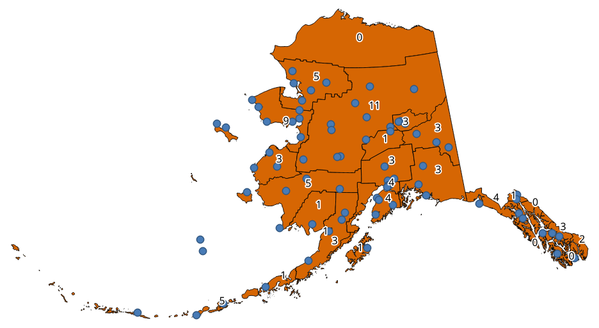Fig. 23.17 The labels in the polygons show the point count

An optional weight field can be used to assign weights to each point. Alternatively, a unique class field can be specified. If both options are used, the weight field will take precedence and the unique class field will be ignored.

`Default menu`: Vector ‣ Analysis Tools

### 23.1.12.3.1. Parameters¶

Label

Nimi

Type

Kuvaus

Polygons

`POLYGONS`

[vector: polygon]

Polygon layer whose features are associated with the count of points they contain

Points

`POINTS`

[vector: point]

Point layer with features to count

Weight field

Optional

`WEIGHT`

[tablefield: any]

A field from the point layer. The count generated will be the sum of the weight field of the points contained by the polygon. If the weight field is not numeric, the count will be `0`.

Class field

Optional

`CLASSFIELD`

[tablefield: any]

Points are classified based on the selected attribute and if several points with the same attribute value are within the polygon, only one of them is counted. The final count of the points in a polygon is, therefore, the count of different classes that are found in it.

Count field name

`FIELD`

[string]

Default: ’NUMPOINTS’

The name of the field to store the count of points

Count

`OUTPUT`

[vector: polygon]

Specification of the output layer

### 23.1.12.3.2. Tulokset¶

Label

Nimi

Type

Kuvaus

Count

`OUTPUT`

[vector: polygon]

Resulting layer with the attribute table containing the new column with the points count

## 23.1.12.4. DBSCAN clustering¶

Clusters point features based on a 2D implementation of Density-based spatial clustering of applications with noise (DBSCAN) algorithm.

The algorithm requires two parameters, a minimum cluster size, and the maximum distance allowed between clustered points.

Katso myös

K-means clustering

### 23.1.12.4.1. Parameters¶

Label

Nimi

Type

Kuvaus

Input layer

`INPUT`

[vector: point]

Layer to analyze

Minimum cluster size

`MIN_SIZE`

[number]

Default: 5

Minimum number of features to generate a cluster

Maximum distance between clustered points

`EPS`

[number]

Default: 1.0

Distance beyond which two features can not belong to the same cluster (eps)

Cluster field name

`FIELD_NAME`

[string]

Default: ’CLUSTER_ID’

Name of the field where the associated cluster number shall be stored

Treat border points as noise (DBSCAN*)

Optional

`DBSCAN*`

[boolean]

Default: False

If checked, points on the border of a cluster are themselves treated as unclustered points, and only points in the interior of a cluster are tagged as clustered.

Clusters

`OUTPUT`

[vector: point]

Vector layer for the result of the clustering

### 23.1.12.4.2. Tulokset¶

Label

Nimi

Type

Kuvaus

Clusters

`OUTPUT`

[vector: point]

Vector layer containing the original features with a field setting the cluster they belong to

Number of clusters

`NUM_CLUSTERS`

[number]

The number of clusters discovered

### 23.1.12.4.3. Python code¶

Algorithm ID: `qgis:dbscanclustering`

```import processing
processing.run("algorithm_id", {parameter_dictionary})
```

The algorithm id is displayed when you hover over the algorithm in the Processing Toolbox. The parameter dictionary provides the parameter NAMEs and values. See Using processing algorithms from the console for details on how to run processing algorithms from the Python console.

## 23.1.12.5. Distance matrix¶

Calculates for point features distances to their nearest features in the same layer or in another layer.

Default menu: Vector ‣ Analysis Tools

### 23.1.12.5.1. Parameters¶

Label

Nimi

Type

Kuvaus

Input point layer

`INPUT`

[vector: point]

Point layer for which the distance matrix is calculated (from points)

Input unique ID field

`INPUT_FIELD`

[tablefield: any]

Field to use to uniquely identify features of the input layer. Used in the output attribute table.

Target point layer

`TARGET`

[vector: point]

Point layer containing the nearest point(s) to search (to points)

Target unique ID field

`TARGET_FIELD`

[tablefield: any]

Field to use to uniquely identify features of the target layer. Used in the output attribute table.

Output matrix type

`MATRIX_TYPE`

[enumeration]

Default: 0

Different types of calculation are available:

• 0 — Linear (N * k x 3) distance matrix: for each input point, reports the distance to each of the k nearest target points. The output matrix consists of up to k rows per input point, and each row has three columns: InputID, TargetID and Distance.

• 1 — Standard (N x T) distance matrix

• 2 — Summary distance matrix (mean, std. dev., min, max): for each input point, reports statistics on the distances to its target points.

Use only the nearest (k) target points

`NEAREST_POINTS`

[number]

Default: 0

You can choose to calculate the distance to all the points in the target layer (0) or limit to a number (k) of closest features.

Distance matrix

`OUTPUT`

[vector: point]

### 23.1.12.5.2. Tulokset¶

Label

Nimi

Type

Kuvaus

Distance matrix

`OUTPUT`

[vector: point]

Point (or MultiPoint for the ”Linear (N * k x 3)” case) vector layer containing the distance calculation for each input feature. Its features and attribute table depend on the selected output matrix type.

### 23.1.12.5.3. Python code¶

Algorithm ID: `qgis:distancematrix`

```import processing
processing.run("algorithm_id", {parameter_dictionary})
```

The algorithm id is displayed when you hover over the algorithm in the Processing Toolbox. The parameter dictionary provides the parameter NAMEs and values. See Using processing algorithms from the console for details on how to run processing algorithms from the Python console.

## 23.1.12.6. Distance to nearest hub (line to hub)¶

Creates lines that join each feature of an input vector to the nearest feature in a destination layer. Distances are calculated based on the center of each feature.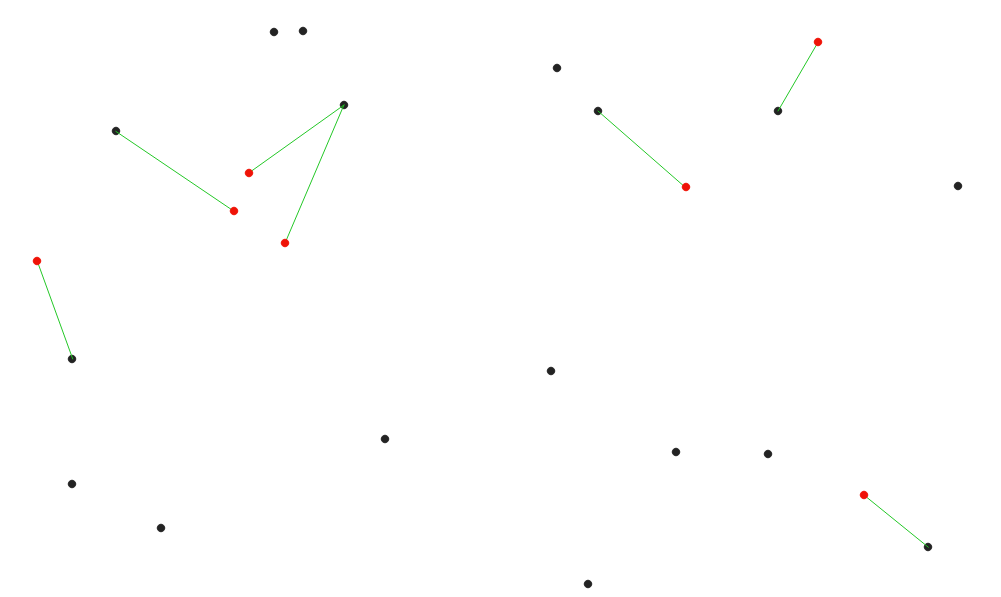Fig. 23.18 Display the nearest hub for the red input features

### 23.1.12.6.1. Parameters¶

Label

Nimi

Type

Kuvaus

Source points layer

`INPUT`

[vector: any]

Vector layer for which the nearest feature is searched

Destination hubs layer

`HUBS`

[vector: any]

Vector layer containing the features to search for

Hub layer name attribute

`FIELD`

[tablefield: any]

Field to use to uniquely identify features of the destination layer. Used in the output attribute table

Measurement unit

`UNIT`

[enumeration]

Default: 0

Units in which to report the distance to the closest feature:

• 0 — Meters

• 1 — Feet

• 2 — Miles

• 3 — Kilometers

• 4 — Layer units

Hub distance

`OUTPUT`

[vector: line]

Line vector layer for the distance matrix output

### 23.1.12.6.2. Tulokset¶

Label

Nimi

Type

Kuvaus

Hub distance

`OUTPUT`

[vector: line]

Line vector layer with the attributes of the input features, the identifier of their closest feature and the calculated distance.

### 23.1.12.6.3. Python code¶

Algorithm ID: `qgis:distancetonearesthublinetohub`

```import processing
processing.run("algorithm_id", {parameter_dictionary})
```

The algorithm id is displayed when you hover over the algorithm in the Processing Toolbox. The parameter dictionary provides the parameter NAMEs and values. See Using processing algorithms from the console for details on how to run processing algorithms from the Python console.

## 23.1.12.7. Distance to nearest hub (points)¶

Creates a point layer representing the center of the input features with the addition of two fields containing the identifier of the nearest feature (based on its center point) and the distance between the points.

### 23.1.12.7.1. Parameters¶

Label

Nimi

Type

Kuvaus

Source points layer

`INPUT`

[vector: any]

Vector layer for which the nearest feature is searched

Destination hubs layer

`HUBS`

[vector: any]

Vector layer containing the features to search for

Hub layer name attribute

`FIELD`

[tablefield: any]

Field to use to uniquely identify features of the destination layer. Used in the output attribute table

Measurement unit

`UNIT`

[enumeration]

Default: 0

Units in which to report the distance to the closest feature:

• 0 — Meters

• 1 — Feet

• 2 — Miles

• 3 — Kilometers

• 4 — Layer units

Hub distance

`OUTPUT`

[vector: point]

Point vector layer for the distance matrix output.

### 23.1.12.7.2. Tulokset¶

Label

Nimi

Type

Kuvaus

Hub distance

`OUTPUT`

[vector: point]

Point vector layer with the attributes of the input features, the identifier of their closest feature and the calculated distance.

### 23.1.12.7.3. Python code¶

Algorithm ID: `qgis:distancetonearesthubpoints`

```import processing
processing.run("algorithm_id", {parameter_dictionary})
```

The algorithm id is displayed when you hover over the algorithm in the Processing Toolbox. The parameter dictionary provides the parameter NAMEs and values. See Using processing algorithms from the console for details on how to run processing algorithms from the Python console.

## 23.1.12.8. Join by lines (hub lines)¶

Creates hub and spoke diagrams by connecting lines from points on the Spoke layer to matching points in the Hub layer.

Determination of which hub goes with each point is based on a match between the Hub ID field on the hub points and the Spoke ID field on the spoke points.

If input layers are not point layers, a point on the surface of the geometries will be taken as the connecting location.

Optionally, geodesic lines can be created, which represent the shortest path on the surface of an ellipsoid. When geodesic mode is used, it is possible to split the created lines at the antimeridian (±180 degrees longitude), which can improve rendering of the lines. Additionally, the distance between vertices can be specified. A smaller distance results in a denser, more accurate line.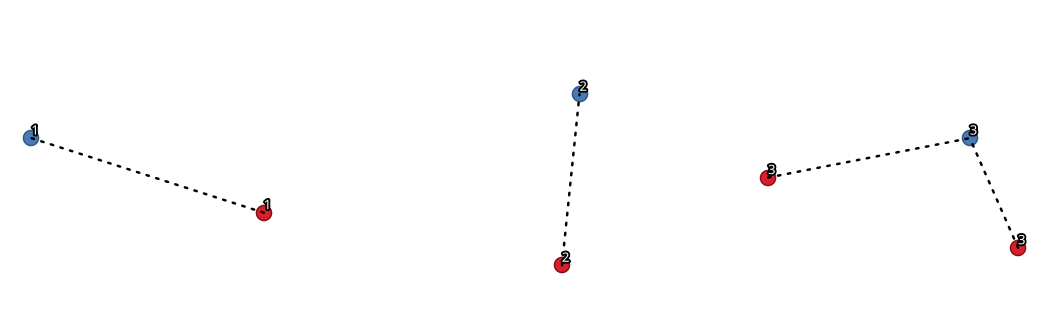Fig. 23.19 Join points based on a common field / attribute

### 23.1.12.8.1. Parameters¶

Label

Nimi

Type

Kuvaus

Hub layer

`HUBS`

[vector: any]

Input layer

Hub ID field

`HUB_FIELD`

[tablefield: any]

Field of the hub layer with ID to join

Hub layer fields to copy (leave empty to copy all fields)

Optional

`HUB_FIELDS`

[tablefield: any] [list]

The field(s) of the hub layer to be copied. If no field(s) are chosen all fields are taken.

Spoke layer

`SPOKES`

[vector: any]

Spoke ID field

`SPOKE_FIELD`

[tablefield: any]

Field of the spoke layer with ID to join

Spoke layer fields to copy (leave empty to copy all fields)

Optional

`SPOKE_FIELDS`

[tablefield: any] [list]

Field(s) of the spoke layer to be copied. If no fields are chosen all fields are taken.

Create geodesic lines

`GEODESIC`

[boolean]

Default: False

Create geodesic lines (the shortest path on the surface of an ellipsoid)

Distance between vertices (geodesic lines only)

`GEODESIC_DISTANCE`

[number]

Default: 1000.0 (kilometers)

Distance between consecutive vertices (in kilometers). A smaller distance results in a denser, more accurate line

Split lines at antimeridian (±180 degrees longitude)

`ANTIMERIDIAN_SPLIT`

[boolean]

Default: False

Split lines at ±180 degrees longitude (to improve rendering of the lines)

Hub lines

`OUTPUT`

[vector: line]

The resulting line layer

### 23.1.12.8.2. Tulokset¶

Label

Nimi

Type

Kuvaus

Hub lines

`OUTPUT`

[vector: line]

The resulting line layer

### 23.1.12.8.3. Python code¶

Algorithm ID: `qgis:hublines`

```import processing
processing.run("algorithm_id", {parameter_dictionary})
```

The algorithm id is displayed when you hover over the algorithm in the Processing Toolbox. The parameter dictionary provides the parameter NAMEs and values. See Using processing algorithms from the console for details on how to run processing algorithms from the Python console.

## 23.1.12.9. K-means clustering¶

Calculates the 2D distance based k-means cluster number for each input feature.

K-means clustering aims to partition the features into k clusters in which each feature belongs to the cluster with the nearest mean. The mean point is represented by the barycenter of the clustered features.

If input geometries are lines or polygons, the clustering is based on the centroid of the feature.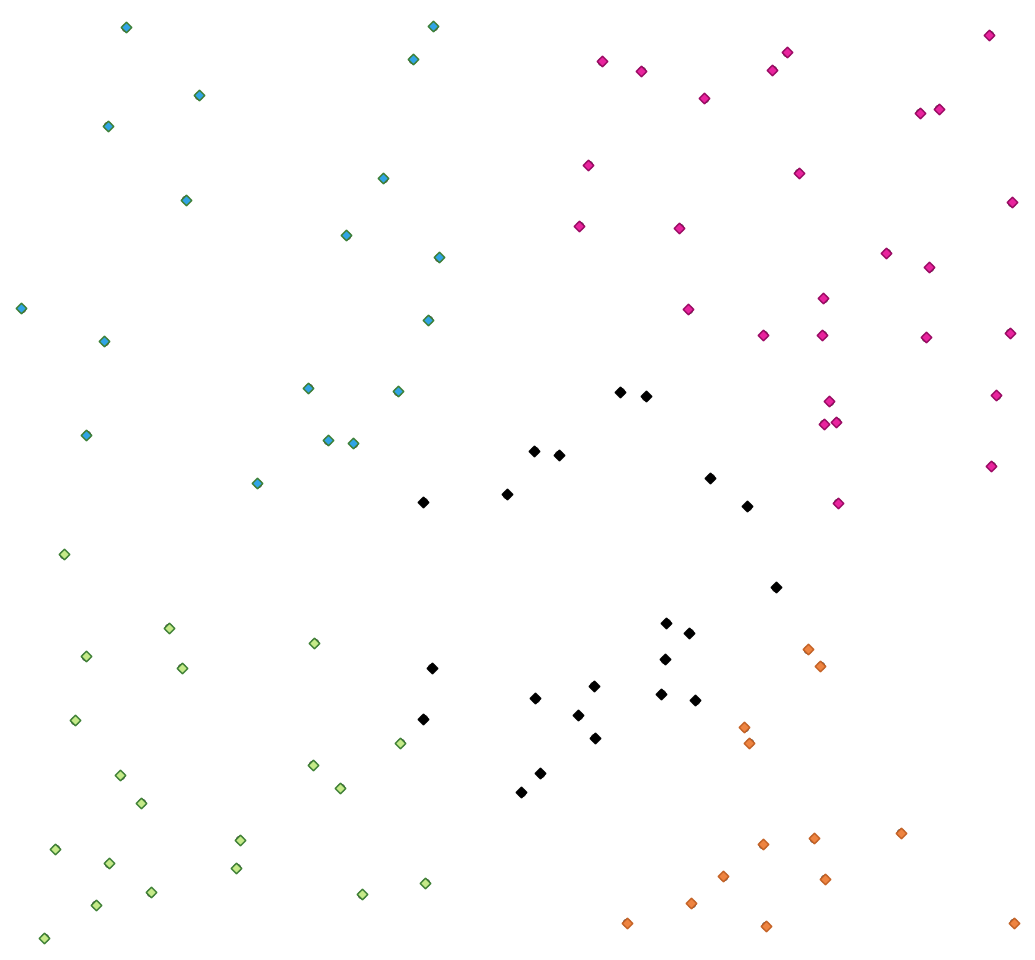Fig. 23.20 A five class point clusters

Katso myös

DBSCAN clustering

### 23.1.12.9.1. Parameters¶

Label

Nimi

Type

Kuvaus

Input layer

`INPUT`

[vector: any]

Layer to analyze

Number of clusters

`CLUSTERS`

[number]

Default: 5

Number of clusters to create with the features

Cluster field name

`FIELD_NAME`

[string]

Default: ’CLUSTER_ID’

Name of the cluster number field

Clusters

`OUTPUT`

[vector: any]

Vector layer for generated the clusters

### 23.1.12.9.2. Tulokset¶

Label

Nimi

Type

Kuvaus

Clusters

`OUTPUT`

[vector: any]

Vector layer containing the original features with a field specifying the cluster they belong to

### 23.1.12.9.3. Python code¶

Algorithm ID: `qgis:kmeansclustering`

```import processing
processing.run("algorithm_id", {parameter_dictionary})
```

The algorithm id is displayed when you hover over the algorithm in the Processing Toolbox. The parameter dictionary provides the parameter NAMEs and values. See Using processing algorithms from the console for details on how to run processing algorithms from the Python console.

## 23.1.12.10. List unique values¶

Lists unique values of an attribute table field and counts their number.

Default menu: Vector ‣ Analysis Tools

### 23.1.12.10.1. Parameters¶

Label

Nimi

Type

Kuvaus

Input layer

`INPUT`

[vector: any]

Layer to analyze

Target field(s)

`FIELDS`

[tablefield: any]

Field to analyze

Unique values

`OUTPUT`

[table]

Summary table layer with unique values

HTML report

`OUTPUT_HTML_FILE`

[html]

HTML report of unique values in the Processing ‣ Results viewer

### 23.1.12.10.2. Tulokset¶

Label

Nimi

Type

Kuvaus

Unique values

`OUTPUT`

[table]

Summary table layer with unique values

HTML report

`OUTPUT_HTML_FILE`

[html]

HTML report of unique values. Can be opened from the Processing ‣ Results viewer

Total unique values

`TOTAL_VALUES`

[number]

The number of uniqe values in the input field

UNIQUE_VALUES

`Unique values`

[string]

A string with the comma separated list of unique values found in the input field

### 23.1.12.10.3. Python code¶

Algorithm ID: `qgis:listuniquevalues`

```import processing
processing.run("algorithm_id", {parameter_dictionary})
```

The algorithm id is displayed when you hover over the algorithm in the Processing Toolbox. The parameter dictionary provides the parameter NAMEs and values. See Using processing algorithms from the console for details on how to run processing algorithms from the Python console.

## 23.1.12.11. Mean coordinate(s)¶

Computes a point layer with the center of mass of geometries in an input layer.

An attribute can be specified as containing weights to be applied to each feature when computing the center of mass.

If an attribute is selected in the parameter, features will be grouped according to values in this field. Instead of a single point with the center of mass of the whole layer, the output layer will contain a center of mass for the features in each category.

Default menu: Vector ‣ Analysis Tools

### 23.1.12.11.1. Parameters¶

Label

Nimi

Type

Kuvaus

Input layer

`INPUT`

[vector: any]

Input vector layer

Weight field

Optional

`WEIGHT`

[tablefield: numeric]

Field to use if you want to perform a weighted mean

Unique ID field

`UID`

[tablefield: numeric]

Unique field on which the calculation of the mean will be made

Mean coordinates

`OUTPUT`

[vector: point]

The (point vector) layer for the result

### 23.1.12.11.2. Tulokset¶

Label

Nimi

Type

Kuvaus

Mean coordinates

`OUTPUT`

[vector: point]

Resulting point(s) layer

### 23.1.12.11.3. Python code¶

Algorithm ID: `qgis:meancoordinates`

```import processing
processing.run("algorithm_id", {parameter_dictionary})
```

The algorithm id is displayed when you hover over the algorithm in the Processing Toolbox. The parameter dictionary provides the parameter NAMEs and values. See Using processing algorithms from the console for details on how to run processing algorithms from the Python console.

## 23.1.12.12. Nearest neighbour analysis¶

Performs nearest neighbor analysis for a point layer. The output tells you how your data are distributed (clustered, randomly or distributed).

Output is generated as an HTML file with the computed statistical values:

• Observed mean distance

• Expected mean distance

• Nearest neighbour index

• Number of points

• Z-Score: Comparing the Z-Score with the normal distribution tells you how your data are distributed. A low Z-Score means that the data are unlikely to be the result of a spatially random process, while a high Z-Score means that your data are likely to be a result of a spatially random process.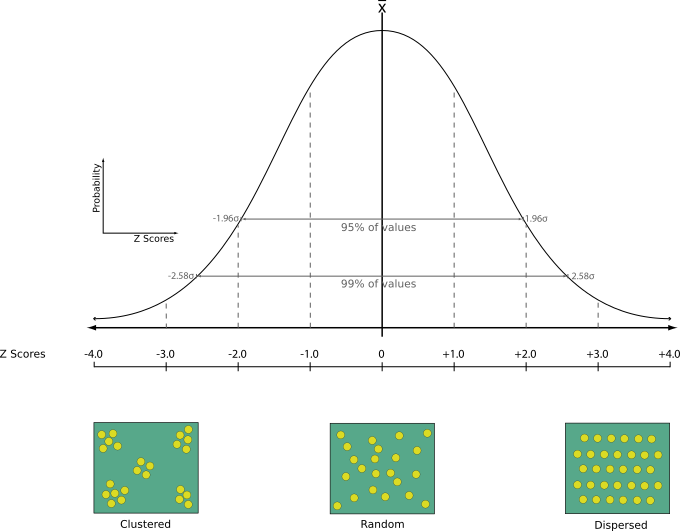Default menu: Vector ‣ Analysis Tools

### 23.1.12.12.1. Parameters¶

Label

Nimi

Type

Kuvaus

Input layer

`INPUT`

[vector: point]

Point vector layer to calculate the statistics on

Nearest neighbour

`OUTPUT_HTML_FILE`

[html]

HTML file for the computed statistics

### 23.1.12.12.2. Tulokset¶

Label

Nimi

Type

Kuvaus

Nearest neighbour

`OUTPUT_HTML_FILE`

[html]

HTML file with the computed statistics

Observed mean distance

`OBSERVED_MD`

[number]

Observed mean distance

Expected mean distance

`EXPECTED_MD`

[number]

Expected mean distance

Nearest neighbour index

`NN_INDEX`

[number]

Nearest neighbour index

Number of points

`POINT_COUNT`

[number]

Number of points

Z-Score

`Z_SCORE`

[number]

Z-Score

### 23.1.12.12.3. Python code¶

Algorithm ID: `qgis:nearestneighbouranalysis`

```import processing
processing.run("algorithm_id", {parameter_dictionary})
```

The algorithm id is displayed when you hover over the algorithm in the Processing Toolbox. The parameter dictionary provides the parameter NAMEs and values. See Using processing algorithms from the console for details on how to run processing algorithms from the Python console.

## 23.1.12.13. Overlap analysis¶

Calculates the area and percentage cover by which features from an input layer are overlapped by features from a selection of overlay layers.

New attributes are added to the output layer reporting the total area of overlap and percentage of the input feature overlapped by each of the selected overlay layers.

### 23.1.12.13.1. Parameters¶

Label

Nimi

Type

Kuvaus

Input layer

`INPUT`

[vector: any]

The input layer.

Overlap layers

`LAYERS`

[vector: any] [list]

The overlay layers.

Output layer

`OUTPUT`

[same as input]

Default: `[Create temporary layer]`

Specify the output vector layer. One of:

• Create Temporary Layer (`TEMPORARY_OUTPUT`)

• Save to File…

• Save to Geopackage…

• Save to PostGIS Table…

The file encoding can also be changed here.

### 23.1.12.13.2. Tulokset¶

Label

Nimi

Type

Kuvaus

Output layer

`OUTPUT`

[same as input]

The output layer with additional fields reporting the overlap (in map units and percentage) of the input feature overlapped by each of the selected layers.

### 23.1.12.13.3. Python code¶

Algorithm ID: `qgis:overlapanalysis`

```import processing
processing.run("algorithm_id", {parameter_dictionary})
```

The algorithm id is displayed when you hover over the algorithm in the Processing Toolbox. The parameter dictionary provides the parameter NAMEs and values. See Using processing algorithms from the console for details on how to run processing algorithms from the Python console.

## 23.1.12.14. Statistics by categories¶

Calculates statistics of a field depending on a parent class. The parent class is a combination of values from other fields.

### 23.1.12.14.1. Parameters¶

Label

Nimi

Type

Kuvaus

Input vector layer

`INPUT`

[vector: any]

Input vector layer with unique classes and values

Field to calculate statistics on (if empty, only count is calculated)

Optional

`VALUES_FIELD_NAME`

[tablefield: any]

If empty only the count will be calculated

Field(s) with categories

`CATEGORIES_FIELD_NAME`

[vector: any] [list]

The fields that (combined) define the categories

Statistics by category

`OUTPUT`

[table]

Table for the generated statistics

### 23.1.12.14.2. Tulokset¶

Label

Nimi

Type

Kuvaus

Statistics by category

`OUTPUT`

[table]

Table containing the statistics

Depending on the type of the field being analyzed, the following statistics are returned for each grouped value:

Statistics

Merkkijono

Numeric

Date

Count (`COUNT`)Unique values (`UNIQUE`)Empty (null) values (`EMPTY`)Non-empty values (`FILLED`)Minimal value (`MIN`)Maximal value (`MAX`)Range (`RANGE`)Sum (`SUM`)Mean value (`MEAN`)Median value (`MEDIAN`)Standard Deviation (`STD_DEV`)Coefficient of variation (`CV`)Minority (rarest occurring value - `MINORITY`)Majority (most frequently occurring value - `MAJORITY`)First Quartile (`FIRSTQUARTILE`)Third Quartile (`THIRDQUARTILE`)Inter Quartile Range (`IQR`)Minimum Length (`MIN_LENGTH`)Mean Length (`MEAN_LENGTH`)Maximum Length (`MAX_LENGTH`)### 23.1.12.14.3. Python code¶

Algorithm ID: `qgis:statisticsbycategories`

```import processing
processing.run("algorithm_id", {parameter_dictionary})
```

The algorithm id is displayed when you hover over the algorithm in the Processing Toolbox. The parameter dictionary provides the parameter NAMEs and values. See Using processing algorithms from the console for details on how to run processing algorithms from the Python console.

## 23.1.12.15. Sum line lengths¶

Takes a polygon layer and a line layer and measures the total length of lines and the total number of them that cross each polygon.

The resulting layer has the same features as the input polygon layer, but with two additional attributes containing the length and count of the lines across each polygon.

Default menu: Vector ‣ Analysis Tools

### 23.1.12.15.1. Parameters¶

Label

Nimi

Type

Kuvaus

Lines

`LINES`

[vector: line]

Input vector line layer

Polygons

`POLYGONS`

[vector: polygon]

Polygon vector layer

Lines length field name

`LEN_FIELD`

[string]

Default: ’LENGTH’

Name of the field for the lines length

Lines count field name

`COUNT_FIELD`

[string]

Default: ’COUNT’

Name of the field for the lines count

Line length

`OUTPUT`

[vector: polygon]

The output polygon vector layer

### 23.1.12.15.2. Tulokset¶

Label

Nimi

Type

Kuvaus

Line length

`OUTPUT`

[vector: polygon]

Polygon output layer with fields of lines length and line count

### 23.1.12.15.3. Python code¶

Algorithm ID: `qgis:sumlinelengths`

```import processing
processing.run("algorithm_id", {parameter_dictionary})
```

The algorithm id is displayed when you hover over the algorithm in the Processing Toolbox. The parameter dictionary provides the parameter NAMEs and values. See Using processing algorithms from the console for details on how to run processing algorithms from the Python console.